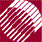ECE532 Biomedical Optics © 1998 Steven L. Jacques, Scott A. Prahl Oregon Graduate Institute

# Time-resolved Monte Carlo

## "trmc.c", a minimal C program

The remainder of this section presents the component parts of a Monte Carlo simulation written in ANSI Standard C, called trmc.c whose source code is listed here.

The program is based on previous work by Steven Jacques, Lihong Wang, Scott Prahl and Marleen Keijzer.

```/********************************************
*  trmc.c    , in ANSI Standard C programing language
*  Time-Resolved Monte Carlo.  Dec. 1998.
*
*  Monte Carlo simulation of time-resolved photon fluence rate
*    in response to an impulse of energy at time zero delivered as an
*    isotropic point source.  Photon propagation is 3D in spherical
*    coordinates. The instantaneous relative fluence rate,
*    F(r,t)/Uo [cm^-2 s^-1]  where F(r,t) = [J cm^-2 s^-1] and Uo = [J].
*    For the calculation, the value of Uo is assumed to be unity [1 J].
*    Propagation is in an infinite homogeneous medium
*    with no boundaries. This program is a minimal Monte Carlo
*    program.
*
*  Results are recorded as F[ir][it]/Uo for each of four timepoints
*    t = T[it], it = 0-3, and 100 radial positions, r = (ir + 0.5)*dr.
*    Results are placed in output file "trmc.out" as columns:
*    r [cm]   F/Uo @ time#1   F/Uo @ time#2   F/Uo @ time#3   F/Uo @ time#4
*
*  by Steven L. Jacques based on prior collaborative work
*    with Lihong Wang, Scott Prahl, and Marleen Keijzer.
*    partially funded by the NIH (R29-HL45045, 1991-1997) and
*    the DOE (DE-FG05-91ER617226, DE-FG03-95ER61971, 1991-1999).
*
**********/
```

After the above verbal description of the program, the header section of the program lists various details necessary for a C program to run. It's not so interesting and you can skip ahead to the next section unless you really want to read the details.

The "include" statements incorporate standard subroutine libraries available with any ANSI standard C compiler.

```#include <math.h>
#include <stdio.h>
```

The "define" statements make global substitutions throughout the program before compiling the program. Hence the progam source code can use simpler names like PI, ALIVE, ONE_MINUS_COSZERO, for easier reading.

A subroutine called RandomGen() is used to generate random numbers between 0 and 1 inclusive. "InitRandomGen" is substituted by a call to RandomGen(0, 1, NULL) which initializes the seed for the random number generator subroutine. "RandomNum" is substituted by a call to RandomGen(1, 0, NULL) which calls for a random number. The function "RandomGen()" is declared, and is listed at the end of the program. In the main program, "InitRandomGen" initializes RandomGen(), and subsequent uses of "RandomNum" calls for a random number from RandomGen().

```#define	PI          3.1415926
#define ALIVE       1   		/* if photon not yet terminated */
#define DEAD        0    		/* if photon is to be terminated */
#define THRESHOLD   0.01		/* used in roulette */
#define CHANCE      0.1  		/* used in roulette */
#define COS90D      1.0E-6
/* If cos(theta) <= COS90D, theta >= PI/2 - 1e-6 rad. */
#define ONE_MINUS_COSZERO 1.0E-12
/* If 1-cos(theta) <= ONE_MINUS_COSZERO, fabs(theta) <= 1e-6 rad. */
/* If 1+cos(theta) <= ONE_MINUS_COSZERO, fabs(PI-theta) <= 1e-6 rad. */
#define SIGN(x)           ((x)>=0 ? 1:-1)
#define InitRandomGen    (double) RandomGen(0, 1, NULL)
/* Initializes the seed for the random number generator. */
#define RandomNum        (double) RandomGen(1, 0, NULL)
/* Calls for a random number from the randum number generator. */

/* DECLARE FUNCTION */
double RandomGen(char Type, long Seed, long *Status);
/* Random number generator */
```

The main program is contained with a section called "main()". Following "main()", the function RandomGen() is listed.

```int main() {

/* PROGRAM SITS WITHIN THIS SECTION */

}
```

NextMonte Carlo